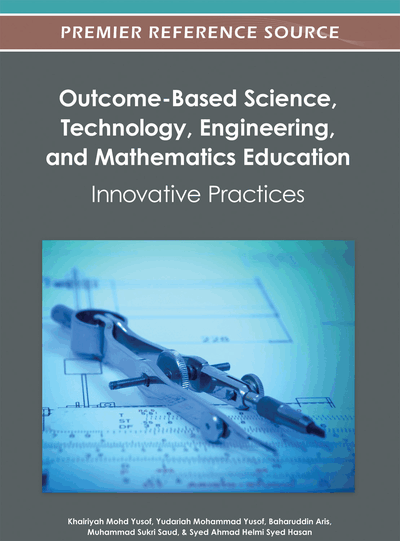# Use of Computer Algebra Systems in Teaching and Learning of Ordinary Differential Equations among Engineering Technology Students

Siti Mistima Maat (Universiti Kuala Lumpur Malaysia France Institute, France) and Effandi Zakaria (Universiti Kebangsaan Malaysia, Malaysia)
DOI: 10.4018/978-1-4666-1809-1.ch010
Available
\$37.50
No Current Special Offers

## Abstract

This chapter describes the computer algebraic system, Maple, used to enhance engineering technology students’ understanding in learning ordinary differential equations (ODE). Ten undergraduate students studying industrial automation and robotics technology were selected to be the participants. They were required to enroll in engineering mathematics as the prerequisite for control system and robotics subject. Engineering mathematics is commonly taught using the traditional approach but the lecturer as a researcher introduced Maple as an alternative teaching approach to engage students in the learning of differential equations. The students were interviewed after using Maple for solving mathematics problems. It was found that these students managed to visualize the solutions of the given mathematics problems. The learning of differential equations has become meaningful in such a way that they could see the relationship between mathematics and its application in the real world.
Chapter Preview
Top

## Introduction

Mathematics and Engineering are two important fields that complement each other in various aspects. A common aspect of these two fields is the focus on engineering mathematics subject. Most engineering problems are classified as applications of mathematics which can be solved using differential equations. It is a challenge for engineering students to explore the relationship between mathematics and engineering fields. Nowadays, finding the solution to the application problem can be obtained easily with computer algebraic systems. Lawson (1997) defined computer algebraic system as a tool which is capable of working symbolically as well as numerically. The integration of mathematics in computer algebraic system can produce solutions of engineering problems that can be visualized with the help of special features. Therefore, the mundane mathematics could be freely explored to be an appreciated subject that everyone likes.

Some common computer algebraic systems like Maple, Mathematica and Matlab have a long list of advantages which have been put forward to make the learning of mathematics more meaningful. By mastering more than one computer algebraic systems, lecturers are able to deliver the subject content effectively. For example, Lua and Yang (1997) reported that Maple helps lecturers to be more creative in preparing teaching materials which can be adjusted according to the students and lecturers’ needs respectively. Integrating Maple in the teaching and learning of mathematics would be beneficial to students in mastering a variety of mathematical concepts used in their field of study (Lua & Yang 1997). Despite the fact that there are many systems available in the market which may be suitable for any discipline; some of them are not suitable for mathematics.

Royrvick (2002) commented that most of the engineering students especially in the electrical field have difficulty in learning engineering subjects due to insufficient competencies in mathematics. The problems include formulating equations to represent the engineering situations. Plugging initial values would be easy for them but judging the correctness of the answers was an issue. This is due to the results in solving problems which used similar formulas from textbooks and had caused misconceptions in learning mathematics. To handle these common situations, Maple has adapted the capabilities of the mathematical software to enhance the students understanding as well as engage them in the learning process. The software could be used to demonstrate difficult topics using its simple program (Latifah, 2006). In addition, Maple provides an exciting atmosphere for complicated mathematical computation as well as graphical representation. The hard and boring work of numerical computation can be removed, leaving students with more time to focus on the concepts and techniques for learning mathematics. This would partially solve the problem of understanding mathematics among students. The students have said that the problem is due to their lack of knowledge on basic mathematical concepts. To learn mathematics, the students need creative and logical thinking. When these problems are combined, more problems will be accumulated that would lead to the difficulty in integrating mathematics and Maple (Latifah, 2006).

## Complete Chapter List

Search this Book:
Reset